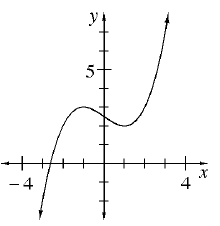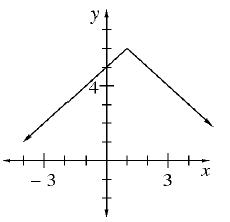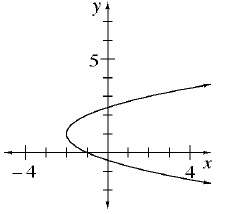### Home > INT1 > Chapter 2 > Lesson 2.1.3 > Problem2-34

2-34.

Which graphs below have a domain of all real numbers? Which have a range of all real numbers? Which are functions?

1.1.1.In which graph do all values of x have a corresponding y-value?
Refer to the Math Notes box in Lesson 1.2.5.

Graphs (a) and (b)

In which graphs do all values of y have a corresponding x-value?

Graphs (a) and (c)

A function can only have one $y$-value for each $x$-value.